#### How to Make Gauss Normal Distribution Chart

In this lesson, you will learn what a gauss chart is and how to insert it. The chart is also known as a normal distribution chart or a bell curve.

The graph of the probability of normal distribution is named after the German mathematician and scientist Johann Gauss. It is he, “Prince of Mathematicians”, who created a feature that allowed the drawing of a bell curve. Today, anyone can create a chart on their own and become the prince of Excel.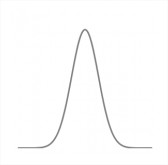The normal distribution, also called the Laplace-Gaussian distribution is one of the most important probability distributions. It plays an important role in the statistical description of the issues of natural, industrial, medical, social, etc. The reason is its prevalence in nature. If a size is the sum or average of a large number of small random factors, regardless of the distribution of each of these factors, the distribution will be close to normal, so it can be very often seen in the data. In addition, the normal distribution has some interesting mathematical properties that make it based on statistical methods computationally simple.

Let’s learn how to create a Gauss chart in Excel.

## Preparing data for a Gauss Chart in Excel

In addition to the mean and standard deviation which are the two basic parameters that describe the normal distribution we need more bands, which allow you to move the desired graph on the horizontal axis. We create two sets of artificial: Series 1 contains the number rising by 0.25 (from -5 to 5), and Series 2 = Series 1 * standard deviation + average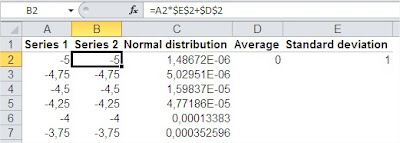Then, in the third column, insert the NORMDIST function, using data from the second series.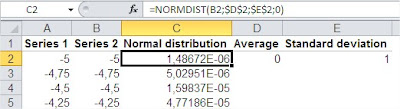## Inserting Gauss Chart in Excel

With so prepared data Select the columns and Series 2 and insert the normal distribution scatter plot with smooth lines. We obtain the following graph.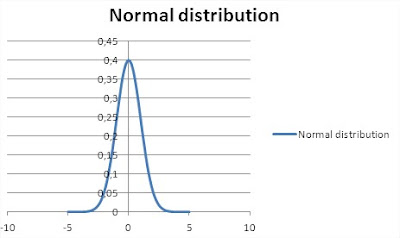After a small correction is made, the beautiful shape of a bell is obtained. It is a Gauss Chart: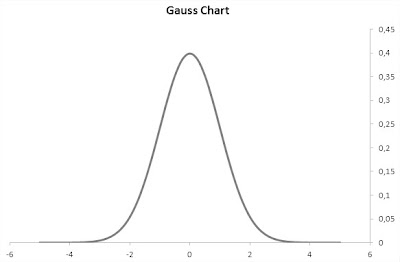Now, changing our input parameters – mean and standard deviation – we can see changes immediately in the chart. Interestingly, the normal distribution perfectly describes the intelligence, height, or intensity of the light source.

## Key Takeaways

• A Gauss chart, also known as a normal distribution chart or a bell curve, is a graph of the probability of normal distribution.
• The normal distribution is one of the most important probability distributions.
• To create a Gauss chart in Excel, you need to prepare data for the chart and then insert the chart.

## FAQ

• Q: What is the difference between a Gauss chart and a histogram?
• A: A Gauss chart is a type of histogram, but it is specifically designed to show the normal distribution. A histogram is a more general-purpose graph that can be used to show any distribution of data.
• Q: What are some other applications of the normal distribution?
• A: The normal distribution is used in a wide variety of fields, including statistics, probability, and engineering. It is also used to model a variety of natural phenomena, such as the height of humans and the IQ of people.